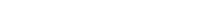# Math riddles Level 33 Answer with Hints and solutionsMath riddles game level 33 Answer with solution android game developed by Black games. Scroll Below to find details.

Math Riddles tests your IQ with mathematical puzzles. Challenge yourself with different levels of math puzzles and stretch the limits of your intelligence. Every IQ game is prepared with an approach of an IQ test. Improve your mathematics, challenge your brain with this game. There are many hard levels which you may not have solved then you can take help from here.

Math app level 33:

3,5=34

8,2=68

4,6=52

5,1=?Solution: the sum of the squares of both the numbers

3×3+5×5=34

8×8+2×2=68

4×4+6×6=52

5×5+1×1=25+1=26

If there is any Doubt/discrepancy then please let me know in comments or you are unable to understand solution then I will surely help you.

### 6 thoughts on “Math Riddles App Level 33 Solution Android”

•December 8, 2019 at 5:38 pm

Why not 28?

First, 11*(3)+7*(5)-34=33+35-34=34
Two, 11*(8)+7*(2)-34=88+14-34=68
Three, 11*(4)+7*(6)-34=44+42-34=52

So, 11*(5)+7*(1)-34=55+7-34=28
My logic also works…
A linear relation.

•February 16, 2019 at 6:57 pm

Why 26 ?
First 3×5=15+15=30+4=34
Two 8×2=16+16=32+32=64+4=68
Three 4×6=24+24=48+4=52
Four 5×1=5+5=10+4=14 or 10+10=20+4=24

I dont understand logic, help pls )

•February 16, 2019 at 11:51 pm

You logic is incorrect as
second should be 32+4=36 but somwhow you again doubled and gave answer.
Detailed logic is now adden to the post itself.

•March 17, 2019 at 8:46 am

The squares of both the numbers, then sum up.

•March 19, 2019 at 12:34 am

Its the SQUARE of both numbers.
I.e 3×3=9 and 5×5=25.

You then add the 25 and 9

•